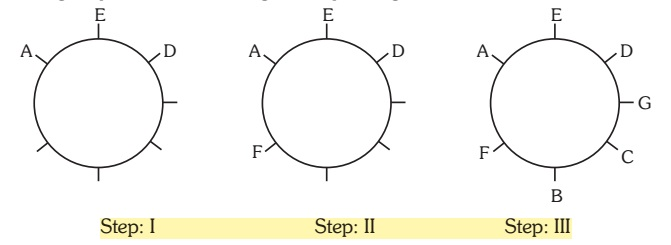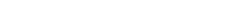## Circular Arrangement

#### Circular Arrangement

(i) A, B, C, D, E, F, and G are sitting along a circle facing at the center and are playing cards,
(ii) E is the neighbor of A and D
(iii) G is not between F and C
(iv) F is on the immediate right of A

1. Who are the neighbor of B?
1. C and D
2. F and C
3. A and F
4. Data inasdequate
5. None of these

1. From the second point we can draw the figure below the position of A and D can interchange but the figures below can be drawn on following the position of F.

##### Correct Option: B

From the second point we can draw the figure below the position of A and D can interchange but the figures below can be drawn on following the position of F. Following the rest points figures are drawn below.1. Which pair given below has the second person sitting immediatley to the right of the first?
1. CB
2. DG
3. EA
4. AB
5. None of these

1. From the second point we can draw the figure below the position of A and D can interchange but the figures below below can be drawn on following the position of F.##### Correct Option: C

EA
From the second point we can draw the figure below the position of A and D can interchange but the figures below below can be drawn on following the position of F. Following the rest points figures are drawn below.1. Which of the following has the persons sitting adjacent to each other left to right in order as given?
1. CDG
2. EDG
3. BGC
4. FBC
5. None of these

1. From the second point we can draw the figure below the position of A and D can interchange but the figures below below can be drawn on following the position of F.##### Correct Option: D

From the second point we can draw the figure below the position of A and D can interchange but the figures below below can be drawn on following the position of F. Following the rest points figures are drawn below.1. What is the position of F?
1. To the immediate left of A
2. To the immediate right of B
3. 2nd to immediate left of C
4. 3rd to the left of D
5. None of these

1. From the second point we can draw the figure below the position of A and D can interchange but the figures below below can be drawn on following the position of F.##### Correct Option: E

From the second point we can draw the figure below the position of A and D can interchange but the figures below below can be drawn on following the position of F. Following the rest points figures are drawn below.1. Which of the following does not have have the pair sitting adjacent to each other?
1. BA
2. CB
3. DE
4. GD
5. All are sitting adjacent to each other.

1. From the second point we can draw the figure below the position of A and D can interchange but the figures below below can be drawn on following the position of F.##### Correct Option: A

From the second point we can draw the figure below the position of A and D can interchange but the figures below below can be drawn on following the position of F. Following the rest points figures are drawn below.